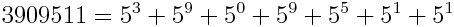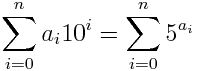# Monday morning math puzzle

This morning Futility Closet mentioned thatHow common is this? In other words, what is the probability that a random set of digits ai will satisfy the following?How does the probability depend on n?

## 9 thoughts on “Monday morning math puzzle”

1. Don’t you mean a sequence of digits a_i, with length n? Python seems to say that nothing below 5000000 satisfies it besides 3909511, though I could be wrong.

2. Pete Krawczyk

The probability is much higher if you do not exclude leading zeroes, for example: 000010. For the sake of the exercise, we will ignore these numbers.

Any number of n digits that contains z zeroes must end in (5*(n-z) + z) modulo 10; that is, for 3909511, which has 7 numbers (1 zero), it must end in 1, because (5*6+1)%10 = 1.

Also, no two-digit number may contain a digit of 3 or above; three-digit, 5+; four-digit, 6+; five-digit: 8+; six-digit numbers may not have a 9. This is because raising 5 to the power of any of the forbidden numbers results in a sum which exceeds the number under consideration. In practice, this rule is unnecessary because 3909511 is the smallest number for which this equation is true.

On the flip side: The maximal number space exists once the value of i is found that satisfies this equation: (10^(i+1))-1 > i*5^9. This equation is true for i=7 but false for i=8; thus, an 8-digit number would be the highest number which could satisfy the equation. Further thinking: The maximal 8-digit number of 99,999,999 gives a numerical sum, as above, of 8*(5^9) – 15,625,000. Ergo, no number larger than 15,625,000 would need to be examined to be true. A nine-digit number would have a maximal numeric sum of 9*(5^9) – 17,578,125 — and as such, no sum could ever get to the 9 digits required to match.

By writing a program in your language of choice (mine is Perl), an exhaustive scan of this number space is trivial — and discovers that 3905911 is the only number which meets this criteria.

3. Pete Krawczyk

…and of course, when I said “true for i=7 but false for i=8”, I meant the exact opposite.

4. Stuart Buck

And how does he come up with similar examples so often?

5. Or more generally for x rather than just 5.

6. Ah, here it is; the answer, unless I’m figuring/pythoning wrong, is pretty boring, did you know it already?

No 8-digit numbers have this property. Beyond that:
5**9 * 9 = 17578125, an 8-digit number. Oh well.

7. 5^9 *7 = 13671875 > 9999999
5^9 *8 = 15625000 < 99999999
5^9 *9 = 17578125 < 999999999

Geometric progression won against arithmetic.

8. Jan Kaluza

John’s original posting got me thinking broader, as Scott commented above, for bases other than just powers of 5. A little Python later I am running a more general form of the problem. This may open a whole new can of worms…

For example: 1^1 = 1
and I guess, technically also: 0^0 = 0
So far I have found the following:

1 is valid for base 1 ( 1^1 = 1 )
none for base 2
12 is valid for base 3 ( 3^1 + 3^2 = 12 )
4624 is valid for base 4
595968 is valid for base 4 (bit of a surprise finding a 2nd number)
3909511 is valid for 5 (validating my method and code)
none for base 6
13177388 is valid for base 7
1033 is valid for base 8

I’ll have to leave it to someone else to get a PhD working further with this idea :)

9. Jan Kaluza

1/2 a day later…. all I found was one more:

10 is valid for base 9

Other than that, we may be looking at a closed set of numbers with this behaviour.